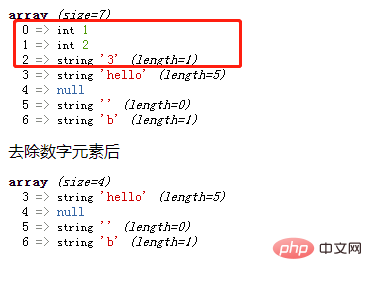# php数组怎么去除数字元素php入门到就业线上直播课：进入学习

```foreach (\$array as \$k => \$v){
//循环体语句块;
}```
• 遍历给定的 \$arr 数组，在每次循环中会将当前数组的值赋给 \$v，键名赋给 \$k。

• is_numeric() 函数用于检测变量是否为数字或数字字符串。如果指定的变量是数字和数字字符串则返回 TRUE，否则返回 FALSE，注意浮点型返回 1，即 TRUE。

• unset() 函数用于销毁给定的变量。

```if (is_numeric(\$v)) {
unset(\$arr[\$k]);
}```

```<?php
function f(\$arr) {
foreach (\$arr as \$k => \$v) {
if (is_numeric(\$v)) {
unset(\$arr[\$k]);
}
}
echo "去除数字元素后";
var_dump(\$arr);
}

\$arr = array(1,2,"3","hello",null,'',"b");
var_dump(\$arr);
f(\$arr);
?>```1/1